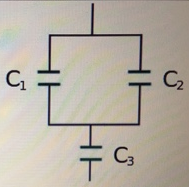# Problem: A combination of series and parallel connections of capacitors is depicted in the figure. C1 = 18 μFC2 = 6.09 μFC3 = 1.85 μFFind the total capacitance of the combination of capacitors, in microfarads.

###### FREE Expert Solution

Capacitors in series:

$\overline{){{\mathbf{C}}}_{\mathbf{e}\mathbf{q}}{\mathbf{=}}\frac{{\mathbf{C}}_{\mathbf{1}}{\mathbf{C}}_{\mathbf{2}}}{{\mathbf{C}}_{\mathbf{1}}\mathbf{+}{\mathbf{C}}_{\mathbf{2}}}}$

In parallel:

$\overline{){{\mathbf{C}}}_{{\mathbf{eq}}}{\mathbf{=}}{{\mathbf{C}}}_{{\mathbf{1}}}{\mathbf{+}}{{\mathbf{C}}}_{{\mathbf{2}}}}$

C1 and C2 are in parallel,

82% (131 ratings)###### Problem Details

A combination of series and parallel connections of capacitors is depicted in the figure.C1 = 18 μF

C2 = 6.09 μF

C3 = 1.85 μF

Find the total capacitance of the combination of capacitors, in microfarads.